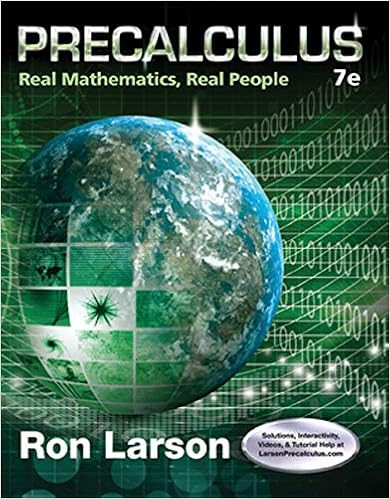# Since the eigenvalues of a hermitian matrix are real

• Notes
• 124
• 100% (3) 3 out of 3 people found this document helpful

This preview shows page 93 - 96 out of 124 pages.

##### We have textbook solutions for you!
The document you are viewing contains questions related to this textbook.The document you are viewing contains questions related to this textbook.
Chapter 7 / Exercise 68
Precalculus: Real Mathematics, Real People
LarsonExpert Verified
Since the eigenvalues of a Hermitian matrix are real, we see that the eigenval- ues of a skew-Hermitian matrix are imaginary.
##### We have textbook solutions for you!
The document you are viewing contains questions related to this textbook.The document you are viewing contains questions related to this textbook.
Chapter 7 / Exercise 68
Precalculus: Real Mathematics, Real People
LarsonExpert Verified
Appendix A Fields and vector spaces Fields A field is an algebraic structure K in which we can add and multiply elements, such that the following laws hold: Addition laws (FA0) For any a , b K , there is a unique element a + b K . (FA1) For all a , b , c K , we have a +( b + c ) = ( a + b )+ c . (FA2) There is an element 0 K such that a + 0 = 0 + a = a for all a K . (FA3) For any a K , there exists - a K such that a +( - a ) = ( - a )+ a = 0. (FA4) For any a , b K , we have a + b = b + a . Multiplication laws (FM0) For any a , b K , there is a unique element ab K . (FM1) For all a , b , c K , we have a ( bc ) = ( ab ) c . (FM2) There is an element 1 K , not equal to the element 0 from (FA2), such that a 1 = 1 a = a for all a K . (FM3) For any a K with a = 0, there exists a - 1 K such that aa - 1 = a - 1 a = 1. (FM4) For any a , b K , we have ab = ba . Distributive law (D) For all a , b , c K , we have a ( b + c ) = ab + ac . 89
90 APPENDIX A. FIELDS AND VECTOR SPACES Note the similarity of the addition and multiplication laws. We say that ( K , +) is an abelian group if (FA0)–(FA4) hold. Then (FM0)–(FM4) say that ( K \{ 0 } , · ) is also an abelian group. (We have to leave out 0 because, as (FM3) says, 0 does not have a multiplicative inverse.) Examples of fields include Q (the rational numbers), R (the real numbers), C (the complex numbers), and F p (the integers mod p , for p a prime number). Associated with any field K there is a non-negative integer called its character- istic , defined as follows. If there is a positive integer n such that 1 + 1 + ··· + 1 = 0, where there are n ones in the sum, then the smallest such n is prime. (For if n = rs , with r , s > 1, and we denote the sum of n ones by n · 1, then 0 = n · 1 = ( r · 1 )( s · 1 ) ; by minimality of n , neither of the factors r · 1 and s · 1 is zero. But in a field, the product of two non-zero elements is non-zero.) If so, then this prime number is the characteristic of K . If no such n exists, we say that the characteristic of K is zero. For our important examples, Q , R and C all have characteristic zero, while F p has characteristic p . Vector spaces Let K be a field. A vector space V over K is an algebraic structure in which we can add two elements of V , and multiply an element of V by an element of K (this is called scalar multiplication ), such that the following rules hold: Addition laws (VA0) For any u , v V , there is a unique element u + v V . (VA1) For all u , v , w V , we have u +( v + w ) = ( u + v )+ w . (VA2) There is an element 0 V such that v + 0 = 0 + v = av for all v V . (VA3) For any v V , there exists - v V such that v +( - v ) = ( - v )+ v = 0. (VA4) For any u , v V , we have u + v = v + u . Scalar multiplication laws (VM0) For any a K , v V , there is a unique element av V . (VM1) For any a K , u , v V , we have a ( u + v ) = au + av .
•••